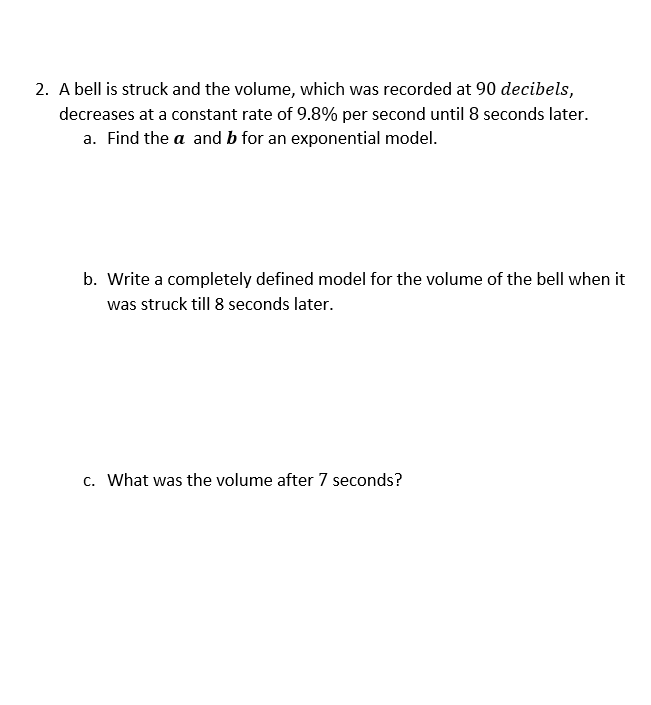2. A bell is struck and the volume, which was recorded at 90 decibels,decreases at a constant rate of 9.8% per second until 8 seconds latera. Find the a and b for an exponential modelb. Write a completely defined model for the volume of the bell when itwas struck till 8 seconds later.c. What was the volume after 7 seconds?

Questionhelp_outlineImage Transcriptionclose2. A bell is struck and the volume, which was recorded at 90 decibels, decreases at a constant rate of 9.8% per second until 8 seconds later a. Find the a and b for an exponential model b. Write a completely defined model for the volume of the bell when it was struck till 8 seconds later. c. What was the volume after 7 seconds? fullscreen
Step 1

a= initial sound= 90 decibels

It decreases 9.8% per second. 9.8%=0.098

Sp after 1 second sound is (1-0.098)=0.902 times of the previous bell.

b= 0.902

Answer(a): a=90 and b=0.902

Step 2

when it strucks till 8 seconds then 0 <= t <=8.

An...

Want to see the full answer?

See Solution

Want to see this answer and more?

Our solutions are written by experts, many with advanced degrees, and available 24/7

See Solution
Tagged in

Functions